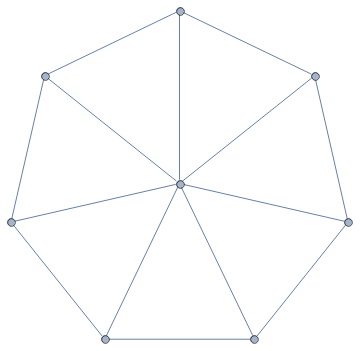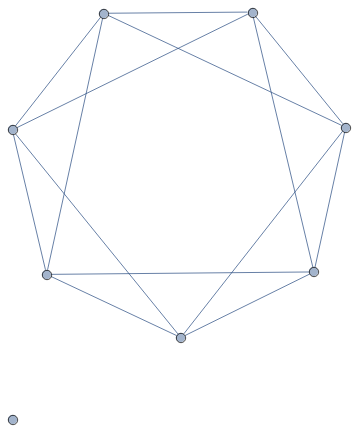## 3-Symmetric Graphs

In my previous post I described 3-symmetric permutations. Now I want to define 3-symmetric graphs.

A reminder: a k-symmetric permutation is such that the densities of all permutations of size k in it are the same. In particular a 2-symmetric permutation has the same number of inversions and non-inversions. How do we translate this to graphs? I call a graph 2-symmetric if it has the same number of edges as non-edges. 2-symmetric graphs are easy to construct, but they only exist if the number of vertices, n, is such that n choose 2 is even. The simplest non-trivial examples are graphs with 4 vertices and three edges.

The above definition is difficult to generalize. So I rephrase: a graph G is 2-symmetric, if the density of any subgraph H with 2 vertices in G is the same as the expected density of H in a random graph where the probability of an edge equals 1/2. This definition is easy to generalize. A graph G is k-symmetric, if the density of any subgraph H with k vertices in G is the same as the expected density of H in a random graph where the probability of an edge equals 1/2. In particular, here are the densities of all four possible subgraphs with 3 vertices in a 3-symmetric graph:

• A complete graph with 3 vertices: 1/8,
• A path graph with 3 vertices: 3/8,
• A graph with 3 vertices and only one edge: 3/8,
• A graph with 3 isolated vertices: 1/8.

For a graph G to be 3-symmetric, the number of vertices, n, in G needs to be such that n choose 3 is divisible by 8. The first non-trivial case is n = 8. Here are the pictures of two 3-symmetric graphs. The first one is a wheel, and the second one is its complement.Share:

### One Comment

1. #### Drake Thomas:

I like both of these definitions of symmetry! I think we can generalize this sort of notion to a wide variety of objects, and prove a nice theorem about their properties.

Suppose we have some probability distribution over objects of a given order, and a way of randomly selecting sub-objects of a given object of some fixed order. Suppose we also have some equivalence relation on these objects. Then we say that an object O is n-symmetric if the distribution over equivalence classes given by choosing a random order-n subobject of O is the same as the one given by choosing a random order-n object.

So in the case of permutations, our order is length, our distributions are the uniform ones, our sub-object relation is to choose a uniformly random subset of n elements in the partition, and our equivalence relation is that of having the same order type. In the case of graphs, our order is number of vertices, our distributions are the ones given by choosing edges with probability 0.5, our sub-object relation is to choose the subgraph induced by a uniformly random order-n subset of the vertices, and our equivalence relation is having the same number of vertices and edges.

Proposition: Suppose that for all k≤n≤m the following 2 conditions hold:

(1) The distribution over equivalence classes given by choosing a random order-k subobject of a random order-n object is the same as the one given by choosing a random element of K.

(2) The distribution over equivalence classes given by choosing a random order-k subobject of a random order-n subobject of a given order-m object O is the same as the one given by choosing a random order-k subobject of O.

Then every n-symmetric object is also a k-symmetric object for all k≤n.

Proof: Let k≤n≤m, and let K,N,M be the sets of all objects of orders k,n,m respectively. Let S be an n-symmetric element of M. Then we have equality between the following distributions (with respect to the chosen equivalence):

[random order-k subobject in S] = [random order-k subobject of (random order-n subobject of S)] = [random order-k subobject of (random element of N)] = [random element of K]

which is exactly the condition for S to be k-symmetric.

Some other objects which can have symmetry defined in this way and satisfy the given conditions under the natural choices for order/equivalence/random object:
* Posets (order = number of elements)
* Directed graphs
* Graphs under other probability distributions (e.g. where a random graph is chosen with edge probability 2/3)
* Words in a finite language with random substrings (not necessarily contiguous). (This doesn’t work if our distribution is contiguous substrings; see below.)

The argument doesn’t hold for every such object – e.g. groups – but it does in most cases where the sub-object relation is “nice” (and in particular when an object’s order counts something, and we can take sub-objects by choosing random subsets of those somethings). (Open question: is there some nontrivial interpretation of random groups and random subgroups which DOES obey these conditions?)

The consequences in each specific case here are nontrivial. For one thing, it restricts our possible orders substantially: the possible orders of 3-symmetric permutations go from 9, 10, 18, 20, … to 9, 20, … and restrict even further for higher orders (the minimal possible orders for 4-symmetric and 5-symmetric permutations go up to 64 and 128, respectively).

An example which is kind of interesting but for which this proposition doesn’t hold: words in a finite language with contiguous substrings. Indeed, BAABB is a 2-symmetric word in the language {A,B} which is not 1-symmetric. The number of 2-symmetric objects has a closed-form solution here: it’s 0 for orders not congruent to 1 mod 4, and at 4k+1 is (2k choose k)^2.

An obvious question for generating n-symmetric graphs: is there any sort of natural operation on a hypercube which yields such graphs? (The motivation being that the complete graph on 2^n vertices always has potential to be n-symmetric.)

I’m not particularly knowledgeable about this, but a friend of mine suggests that this may have connections to the theory of flag algebras (https://arxiv.org/pdf/1607.04741.pdf).